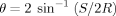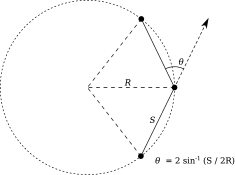# Track step angles?

Dear MRtrix experts -

I am interested in measuring tract tortuosity / curvature.

Is there a way to interogate a .tck file to find the average angle between successive steps… averaged across all tracks?

I would probably want to downsample my tracks and try this at various step lengths to vary the weighting between noise and anatomy. Also, I’d want to normalise to the length of the track. I know how to do these, but just not how to get the step angles.

I couldnt find any info on this. Any advice?

Many thanks - Tom

Hi @twelton,

As far as I know, we’ve got no MRtrix commands that do this straight out of the box. The easiest would probably be to read the `tck` file into Matlab or Python or something similar, where writing a loop over the track, and getting these angles (and averaging) should be quite simple.

As a side note: I reckon the downsampling at different step sizes/lengths will definitely have an impact on such tortuosity or other curvature measures. You may want to consider looking at local radii of curvature instead (which relate the step sizes to these angles, to produce a measure of curvature that is theoretically independent of the step size). See this relationship:

This radius corresponds to the radius of the circle defined by the three vertices of two successive track segments. The relationship between θ, the angle between the two track segments, and R, the radius of curvature, is:where S is the step size.(from this paper: http://onlinelibrary.wiley.com/doi/10.1002/ima.22005/abstract )

Cheers,
Thijs

Tom,

There is some code here for calculating streamline curvature of wiggly probabilistic streamlines. Unfortunately it is currently embedded deep within the Track-Weighted Imaging (TWI) code, and is not directly accessible via the user interface. A future update will expose this functionality at the command-line; but for now, if you have any capability with C++, this code may provide you with a starting point.

Rob

Hi guys,

Thanks for your suggestions. Here’s my MATLAB function. Solvingfor R:

R=S/(2*sin(theta/2))

``````function [ tract_tortuosity ] = tract_tortuosity_radius( track_file )
% return the average step-to-step curvature radius in mm averaged across all tracks

track_count = str2double(tracks.count);
step_size = tracks.step_size;

% for each track in the file
for track_number = 1:track_count
track = tracks.data{1,track_number};
track_step_count = size(track,1);

% for each step in the track
for step_number = 1:track_step_count-2

% get two vectors
point1 = track(step_number,:);
point2 = track(step_number+1,:);
point3 = track(step_number+2,:);

step1 = point2 - point1;
step2 = point3 - point2;

end

end

end
``````

I tested these three tracts for curvature radius (similar numbers of tracks and lengths):

I tested the tracts at step lengths from 1 to 35 mm (downsampled using `tckresample`). Here are the average radii for each tract at various step lengths (colours matching tracts as above):

Here are the radii and angles (in radians) for every track in the blue tract for step length=1 (x-axis is steps):

It looks like the tracts are best distinguished at step lengths > 5mm. For very long step lengths, many details are lost (for example 35mm sampling in the fig below), but the method still seems to work regardless. I think I’ll choose a step length between 5 and 10 mm and use that.

Cheers - Tom

1 Like

Thanks for reporting back with some results. Those are certainly nice insights!

Just for the sake of clarity:

Part of the operation of the code I linked to above is to perform smoothing of streamlines tangents prior to curvature quantification, reducing the effect of streamline ‘squiggliness’. While the extent of smoothing is currently hard-coded, that’s really only an interface issue. Down-sampling streamlines to a larger step size before calculating curvature based on the equation you have used will have a similar effect to modulating the extent of tangent smoothing; but results in your case may vary depending on exactly where the re-sampled streamline vertices happen to lie. The other potential disadvantage of the latter approach is the heavier discretization of number of sample points along each track, which may be detrimental depending on exactly what you’re looking to do with the data subsequently.

If you’re interested in pursuing this kind of quantification more rigorously, I can try to expedite exposing access to the curvature quantification capability in an MRtrix3 command?

Rob

Hi Rob.

I may return to it at some point but, for now, what I have is good enough for my purposes. I do think it would be a nice feature to have in future, though, as a potentially important factor for tracking performance.

Cheers - Tom

1 Like